Question

# We are building a road, and at one place we need to make a turn with...

We are building a road, and at one place we need to make a turn with a radius of 30m.  The coefficient of static friction between rubber and the material we are using is 0.6. Instead of keeping the road flat, let's bank the turn a little bit, giving the road an angle of 20﻿∘ above the horizontal. With the banked turn, what is the maximum speed a car could have and still make it around the turn without sliding?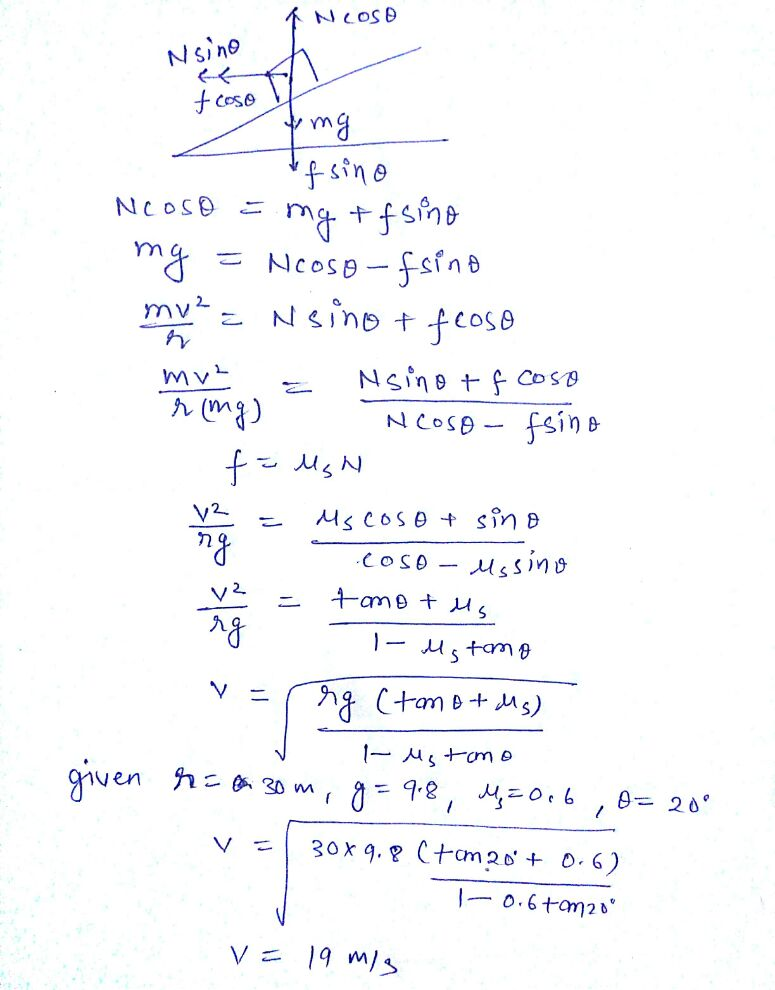#### Earn Coins

Coins can be redeemed for fabulous gifts.

Similar Homework Help Questions
• ### gth of the Cirrl 1. A 1300 kg car moving on a flat, horizontal road negotiates...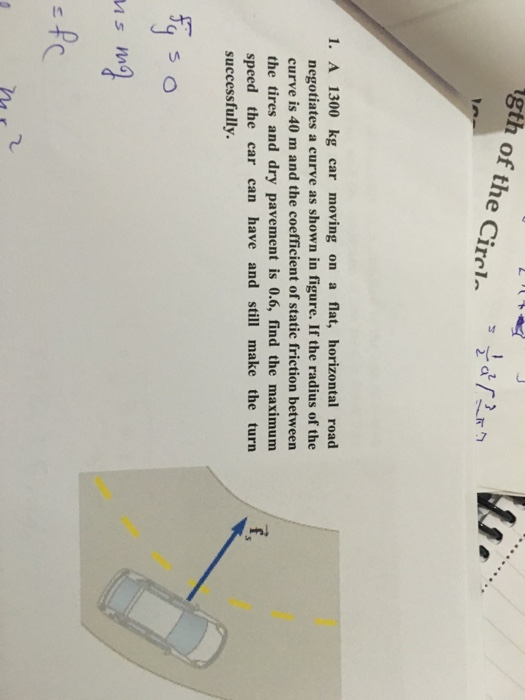gth of the Cirrl 1. A 1300 kg car moving on a flat, horizontal road negotiates a curve as shown in figure. If the radius of the curve is 40 m and the coefficient of static friction between the tires and dry pavement is 0.6, find the maximum speed the car can have and still make the turn successfully.

• ### A car at speed v takes a turn of radius R on a banked road of...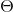A car at speed v takes a turn of radius R on a banked road of angle . What is the angle that the road must be banked ti bit require the driver to turn the steering wheel? For circular motion, the centripetal acceleration is Now the road has a coefficient of friction of with . What is the maximum velocity that the driver can take the turn? We were unable to transcribe this imageWe were unable to transcribe this...

• ### A car travels around a horizontal bend of radius 177 m at a constant speed. (a)...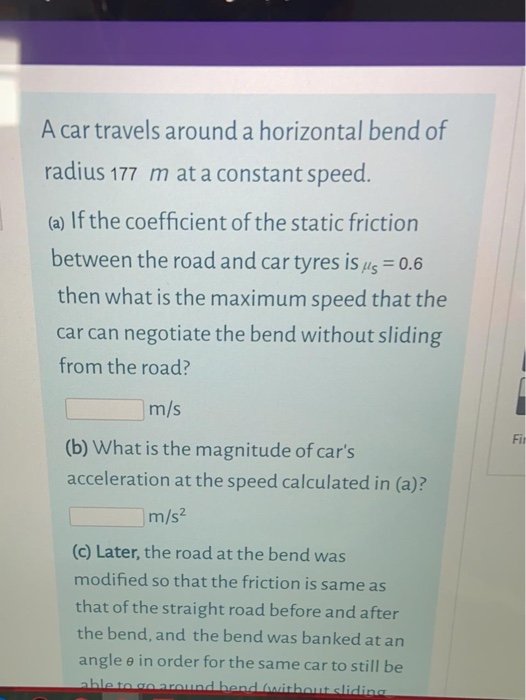A car travels around a horizontal bend of radius 177 m at a constant speed. (a) If the coefficient of the static friction between the road and car tyres is us = 0.6 then what is the maximum speed that the car can negotiate the bend without sliding from the road? m/s Fil (b) What is the magnitude of car's acceleration at the speed calculated in (a)? m/s2 (c) Later, the road at the bend was modified so that the...

• ### You are driving your car along a flat, curved road; the curve in the road is...

You are driving your car along a flat, curved road; the curve in the road is a segment of a circle with radius 50 meters. (We call this a "radius of curvature"). How fast can the car drive around the curve if the coefficient of static friction between the tires and the road is 1.0 (tires on dry pavement)? What if the coefficient of friction is 0.2 (tires on ice)?

• ### A car with mass 444 kg attempts to make a turn with a radius of 27.8...

A car with mass 444 kg attempts to make a turn with a radius of 27.8 m at a speed of 17.5 m/s on a horizontal surface. What is the minimum value for the static coefficient of friction between the tires and the roadway necessary for the car to make the turn without sliding? Give your answer with 3 significant figures.

• ### A car with mass 444 kg attempts to make a turn with a radius of 27.8...

A car with mass 444 kg attempts to make a turn with a radius of 27.8 m at a speed of 17.5 m/s on a horizontal surface. What is the minimum value for the static coefficient of friction between the tires and the roadway necessary for the car to make the turn without sliding? Give your answer with 3 significant figures.

• ### A car of mass M = 800 kg traveling at 55.0 km/hour enters a banked turn...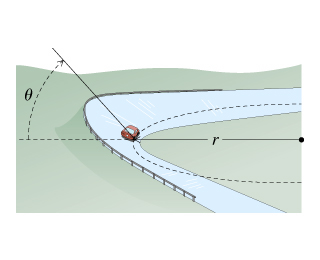A car of mass M = 800 kg traveling at 55.0 km/hour enters a banked turn covered with ice. The road is banked at an angle ?, and there is no friction between the road and the car's tires as shown in(Figure 1) . Use g = 9.80 m/s2 throughout this problem. Now, suppose that the curve is level (?=0) and that the ice has melted, so that there is a coefficient of static friction ? between the road and...

• ### A particular unbanked turn in the road is shaped like a circle with a radius of...

A particular unbanked turn in the road is shaped like a circle with a radius of 30 meters. A car with a mass of 1500 kg can safely go around this turn at a maximum speed of 17 m/s. What is the coefficient of static friction between the car's tires and the road?

• ### PLEASE ANSWER PART B. THANKS! t Banked Frictionless Curve, and Flat Curve with Friction A car...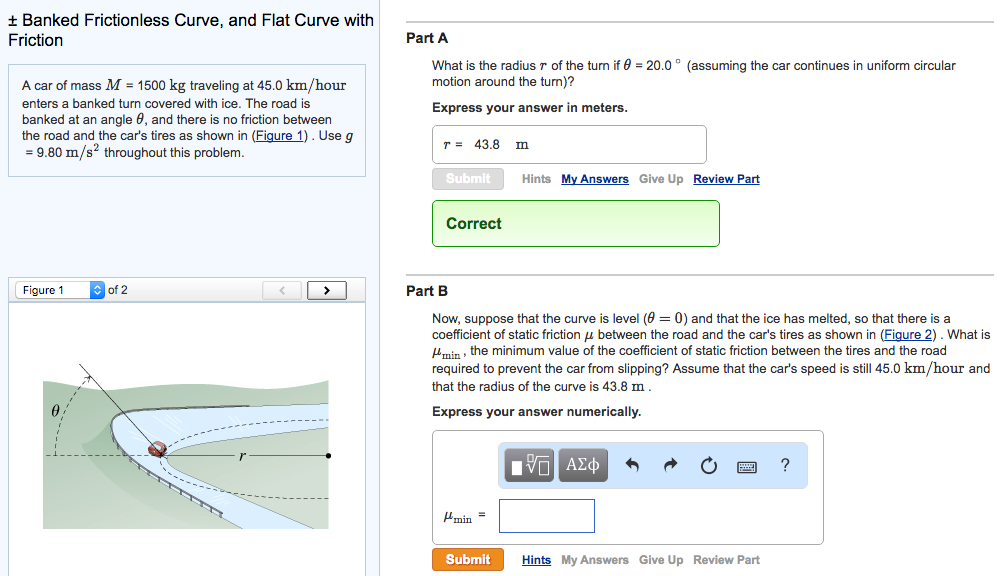PLEASE ANSWER PART B. THANKS! t Banked Frictionless Curve, and Flat Curve with Friction A car of mass M 1500 kg traveling at 45.0 km/hour enters a banked turn covered with ice. The road is banked at an angle 6, and there is no friction between the road and the car's tires as shown in (Figure 1). Use g 9.80 m/s2 throughout this problem. of 2 Figure 1 Part A What is the radius r of the turn if 0...

• ### A car of mass M = 1300 kg traveling at 65.0 km/hour enters a banked turn...

A car of mass M = 1300 kg traveling at 65.0 km/hour enters a banked turn covered with ice. The road is banked at an angle θ, and there is no friction between the road and the car's tires as shown in (Figure 1) . Use g = 9.80 m/s2 throughout this problem. r= 91.43 m. Now, suppose that the curve is level (θ=0) and that the ice has melted, so that there is a coefficient of static friction μ...